Courses

# MCQ: Areas of Parallelograms and Triangles - Class 9 Class 9 Notes | EduRev

## Class 9 : MCQ: Areas of Parallelograms and Triangles - Class 9 Class 9 Notes | EduRev

The document MCQ: Areas of Parallelograms and Triangles - Class 9 Class 9 Notes | EduRev is a part of Class 9 category.
All you need of Class 9 at this link: Class 9

ABC and BDE are two equilateral triangles such that D is the mid point of BC. AE intersects BC in F. Then ar (BDE) is equal to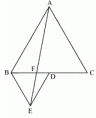• a)
2ar (ABC)
• b)
1/4ar (ABC)
• c)
1/2ar (ABC)
• d)
ar (ABC)
Correct answer is option 'B'. Can you explain this answer?

Ref: https://edurev.in/question/575384/ABC-and-BDE-are-two-equilateral-triangles-such-tha

Given: ABC and BDE are two equilateral triangles such that D is the mid-point of BC. AE intersects BC at F.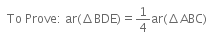Construction: Join EC and AD.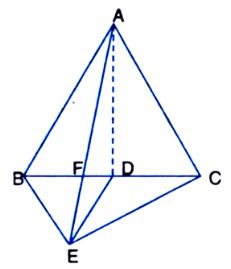Proof: ∵ ΔABC is an equilateral triangle. ∴ ∠ABC = ∠BCA = ∠CAB = 60° ...(1)
∵    ΔBDE is an equilateral triangle.
∴ ∠BDE = ∠DEB = ∠EBD = 60° ...(2) ∠ABE + ∠BED
= ∠ABD + ∠EBD + ∠BED = 60°+ 60°+ 60°= 180°
∴ AB || DE    ...(3)
∵    Sum of consecutive interior angles on
the same side of a transversal is 180° ∠EBA + ∠BAC
= ∠EBD + ∠DBA + ∠BAC = 60° + 60° + 60° = 180°
∴ AC || BE    ...(4)
∵    Sum of consecutive interior angles on the same side of the transversal is 180°
∵    ΔCBA and ΔCEA are on the same base AC and between the same parallels.
∴ ar(ΔCBA) = ar(ΔCEA)
Two triangles on the same base (or equal bases) and between the same parallels are equal in area ⇒ ar(ΔABC) = ar(ΔCDA) + ar(ΔCED) + ar(ΔADE) ...(5)
In ΔABC,
∵    AD is a median.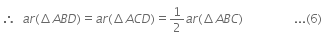∵ A median of a triangle divides it into two triangles of equal area
In ΔEBC,
∵ ED is a median.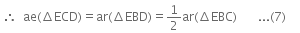∵ A median of a triangle divides it into two triangles of equal area ∵ ΔDEA and ΔDBE are on the same base DE and between the same parallels AB and DE.
∴ ar(ΔDEA) = ar(ΔDBE)    ...(8)
∵ Two triangles on the same base (or equal bases) and between the same parallels are equal in area
Using (6), (7) and (8), (5) gives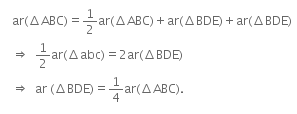Offer running on EduRev: Apply code STAYHOME200 to get INR 200 off on our premium plan EduRev Infinity!

,

,

,

,

,

,

,

,

,

,

,

,

,

,

,

,

,

,

,

,

,

;